SLUS157Q December   1999  – October 2019

PRODUCTION DATA.

1. Features
2. Applications
3. Description
1.     Device Images
4. Revision History
5. Pin Configuration and Functions
6. Specifications
7. Detailed Description
8. Application and Implementation
1. 8.1 Application Information
2. 8.2 Typical Application
1. 8.2.1 Design Requirements
2. 8.2.2 Detailed Design Procedure
3. 8.2.3 Application Curves
9. Power Supply Recommendations
10. 10Layout
11. 11Device and Documentation Support
12. 12Mechanical, Packaging, and Orderable Information

• DW|20
• PW|20
• N|20
• PW|20

#### 8.2.2.10.2 Voltage Loop Compensation

We choose a standard configuration for a TL431 / optocoupler based feedback network. Type 2 loop compensation is appropriate for a design using peak current mode control. First we set the DC operating points for the TL431 (U1) and the optocoupler (U2).

We assume that the optocoupler (U2) has a current transfer ratio (CTR) of 1 and choose to operate it at a maximum LED current, IF of 10 mA. RD is then given by:

Equation 103.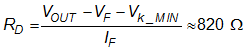We set the parallel combination of RG and RF to 2.4 kΩ for a nominal 10-dB gain for output perturbations via the direct path from RD to the optocoupler diode. This path exists in parallel with the path through RA and the TL431. The direct path is important at frequencies where the gain of the TL431 integrator has fallen to 0 dB.

RG and RF form a potential divider whose function is to keep the EAP pin within the upper limit of its common mode input range (VCM_MAX = 3.6 V) when there is no current in the photo-transistor. RG is connected to VREF and this constraint on the voltage at the EAP pin gives:

Equation 104.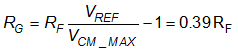Since we know the parallel value of RF and RG and their ratio (RF/RG), we calculate RF as follows:

Equation 105.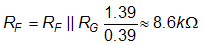and

Equation 106.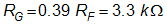At low frequencies the gain is dominated by the response of the TL431 error amplifier which is configured as a pure integrator. The TL431 has a typical open loop gain of about 60 dB at DC, which decreases at the normal –20 dB per decade. Its gain will be 0 dB when the impedance of CE falls to that of RA. Even though the TL431 gain has fallen to 0 dB, the system still has 10-dB gain due to the direct path through RD.

We put the zero due to capacitor CE and resistor RA at the desired 0-dB gain frequency of 2 kHz. Since RA is already selected from VOUT setpoint considerations we calculate CE as follows:

Equation 107.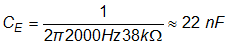The optocoupler has a 10-dB response through the direct path, to perturbations on VOUT. At higher frequencies the capacitance at the collector of the optocoupler (CF) forms a pole with the resistor in series with the optocoupler LED. The gain then rolls off in half a decade to reach 0 dB. With CF = 68 nF this pole is at about 2.8 kHz.

Having chosen the component values in the feedback path around the TL431 we can draw a Bode Plot of the VOUT to EAP transfer function GC(f).

The control to output transfer function of the power train is approximated by:

Equation 108.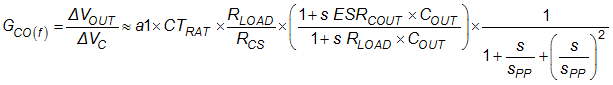where

• s = 2πjf is the complex frequency
• sPP is FSW / 2 = 50 kHz in this case
• The overall loop response is then given by GC(f). GC(o).

This loop response has a crossover frequency of 7.5 kHz. TI recommends that you check the loop stability of the final design with load transient tests and by checking that the gain and phase margins are sufficient. RLOOP provides a convenient point to inject signals for loop gain and phase measurements. The feedback network may need to be adjusted to achieve satisfactory performance.